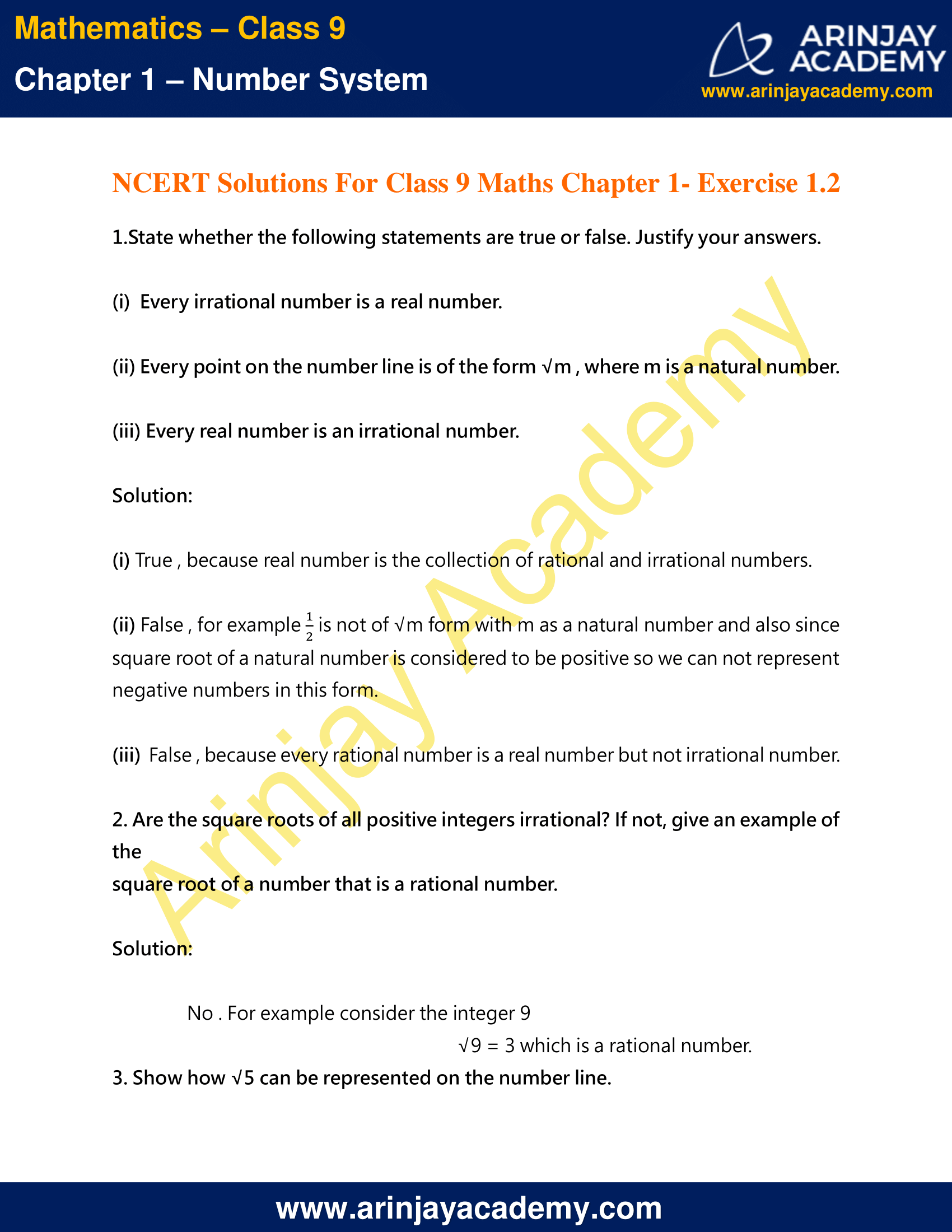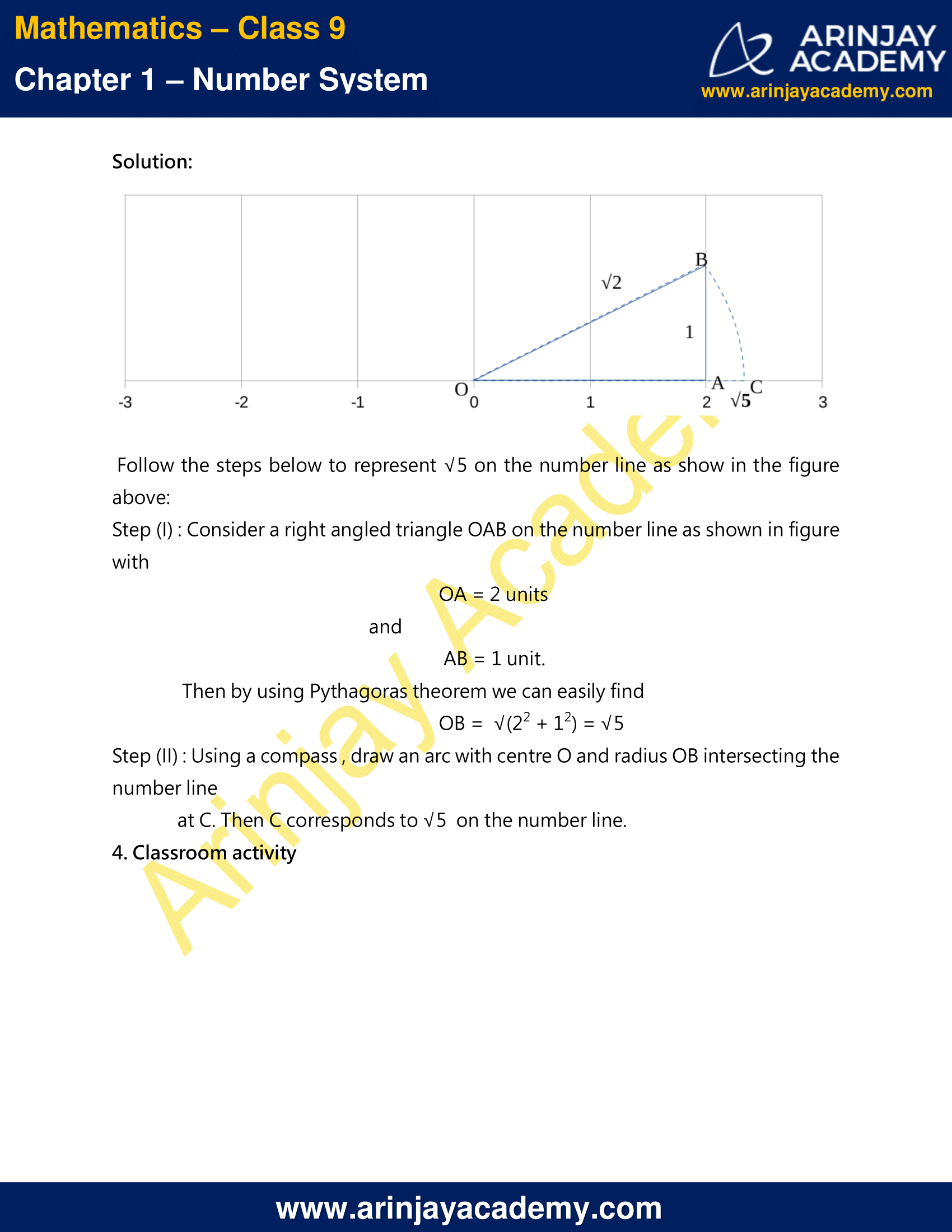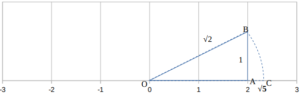# NCERT Solutions For Class 9 Maths Chapter 1 Exercise 1.2 – Number System

Download NCERT Solutions For Class 9 Maths Chapter 1 Exercise 1.2 – Number System. This Exercise contains 4 questions, for which detailed answers have been provided in this note. In case you are looking at studying the remaining Exercise for Class 9 for Maths NCERT solutions for Chapter 1 or other Chapters, you can click the link at the end of this Note.

### NCERT Solutions For Class 9 Maths Chapter 1 Exercise 1.2 – Number SystemNCERT Solutions For Class 9 Maths Chapter 1 Exercise 1.2 – Number System

1.State whether the following statements are true or false. Justify your answers.
(i) Every irrational number is a real number.
(ii) Every point on the number line is of the form √m , where m is a natural number.
(iii) Every real number is an irrational number.

Solution 1

(i) True , because real number is the collection of rational and irrational numbers.
(ii) False , for example 1/2 is not of √m form with m as a natural number and also since square root of a natural number is considered to be positive so we can not represent negative numbers in this form.
(iii) False , because every rational number is a real number but not irrational number.

2. Are the square roots of all positive integers irrational? If not, give an example of the square root of a number that is a rational number.

Solution 2

No, For example consider the integer 9
√9 = 3 which is a rational number

3. Show how √5 can be represented on the number line.

Solution:Follow the steps below to represent √5 on the number line as show in the figure above:

Step (I) : Consider a right angled triangle OAB on the number line as shown in figure with
OA = 2 units
AB = 1 unit.
Then by using Pythagoras theorem we can easily find
OB = √(2² + 1²) = √5
Step (II) : Using a compass , draw an arc with centre O and radius OB intersecting the number line at C. Then C corresponds to √5 on the number line.

4. Classroom activity

NCERT Solutions for Class 9 Maths Chapter 1 Exercise 1.2 – Number System, has been designed by the NCERT to test the knowledge of the student on the topic – Irrational Numbers

The next Exercise for NCERT Solutions for Class 9 Maths Chapter 1 Exercise 1.3 – Number System can be accessed by clicking here.

Download NCERT Solutions For Class 9 Maths Chapter 1 Exercise 1.2 – Number System